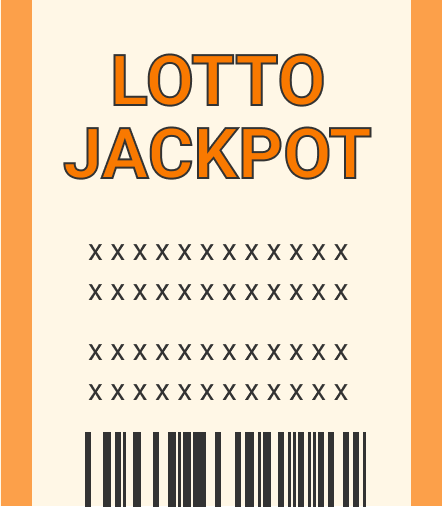### Random Variables & Distributions

In this finale quiz, we'll apply what we know about random variables and probability distributions to real-world problems.

Since these applications are inspired by real-life scenarios, they're more challenging than the problems we looked at in the last two quizzes.

The final problem in particular requires calculus; it may be skipped without loss.

# Random Variable ApplicationsThe display of a certain phone brand gives out at random instants in time at a constant rate of $k$ failures per second. If $X$ is the number of breakdowns in the length of time $t$ since its release, then $X$ is distributed like a Poisson variable, which means: $P(X=n) = \frac{\lambda^{n}}{n!} e^{-\lambda}, \ n = 0 , 1 , 2 , \ldots, \ \ \ \ \lambda = k t$ What is the expected number of failures $E[X] = \sum\limits_{n \in X\text{'s range}} n P(X = n) = \sum\limits_{n=0}^{\infty} n \frac{\lambda^{n}}{n!} e^{-\lambda} \ ?$

Hint: The exponential function is defined by the infinite sum $e^{x} = \sum\limits_{n=0}^{\infty} \frac{x^n}{n!}.$

# Random Variable Applications

We'd also like to know how spread out these failures are from the average. Find the variance $\text{Var}[X] = E\big[X^2\big] - \big( E[X]\big)^2$ for the number of display breakdowns from the last problem, which is distributed as $P(X=n) = \frac{\lambda^{n}}{n!} e^{-\lambda}, \ n = 0 , 1 , 2 , \ldots, \ \ \text{where} \ e^{x} = \sum\limits_{n=0}^{\infty} \frac{x^n}{n!}.$

# Random Variable ApplicationsLet's say a particular trait, like hair or eye color, is determined by one pair of genes. Let D be the dominant gene, and R the recessive.

The possible gene combinations are DD (pure dominance), DR or RD (hybrid), or RR (pure recessive). Children with at least one copy of the dominant gene exhibit the dominant trait.

A child receives one gene from each parent, and assuming they're both hybrids, each of the child's genes is equally likely to be a D or an R.

If a pair of hybrid parents have a total of T children, of which N exhibit the dominant trait (i.e. have the dominant gene), what is $P(N = n ) ?$

Good to Know: The number of ways to choose $k$ objects from a set of $n$ is ${n \choose k} = \frac{n!}{k!(n-k)!},$ where $n! = n(n-1)(n-2) \cdots 1$ for $\ n \geq 1$ and $0! = 1.$

# Random Variable Applications

In a very large sample of families with eight children, what's the average number of children not showing the appearance of the dominant trait?

Hint: For a binomial random variable $P(N=n) = { T \choose n }p^n \left( 1-p \right)^{T-n},\ E[N] = T p.$

# Random Variable Applications

It's reported that the probability of winning a certain state lottery is about $\frac{1}{8}.$ Let $X$ be the number of tickets purchased up to and including the first win.

What is $P( X = k )$ for $k = 1, 2, 3, \dots ?$# Random Variable Applications

What's the probability of winning this lottery in five tries or less?

Good to Know: $\displaystyle \sum\limits_{j=0}^{k} p^{j} = \frac{1-p^{k+1}}{1-p} , \ 0 < p < 1$

# Random Variable Applications

The distribution $P(X=k) = \left( \frac{7}{8} \right)^{k-1} \frac{1}{8}$ is an example of a geometric distribution because of its relationship with the geometric sum $\sum\limits_{j=0}^{\infty} p^{j} = \frac{1}{1-p} , \ 0 < p < 1.$ If the lotto from the last problem pays out $1,000 on a win but costs$1 per ticket, what's the average payout if you play to the first win?

Hint: The expected value of a function of a random variable is $E\big[f(X)\big] = \sum\limits_{ k \in X \text{'s range}} f(k) P(X = k ),$ and $\sum\limits_{j=0}^{\infty} j p^{j} = \frac{p}{(1-p)^2}, \ 0 < p < 1.$

# Random Variable Applications

We found it useful to assign distribution functions to continuous random variables: $P( a \leq X \leq b) = \int\limits_{x = a }^{x=b} f(x) dx$ for some density function $f(x).$ For instance, the time $t$ it takes for the decay of a radioactive carbon-14 atom into stable nitrogen-14 is distributed exponentially, which means: $f(t) = a e^{-a t} , \ t \geq 0,$ where $a>0$ is a constant. What's the probability that a carbon-14 atom lasts at least as long as $\frac{1}{a} ?$

# Random Variable Applications

In this quiz, we sampled some applications of continuous and discrete random variables and their probability distributions.

The rest of the course will explore these ideas and other applications in greater depth.

# Random Variable Applications

×